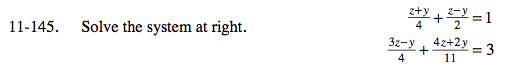### Home > A2C > Chapter Ch11 > Lesson 11.3.2 > Problem11-145

11-145.In the first equation, find the least common denominator and simplify.

z + y + 2z − 2y = 4

Now solve for y.

Use the same process for the second equation.

Substitute the value you found for y into the answer from the second hint.

y = 5 z = 3